### 8.29. Border Average

#### 8.29.1. Overview

Figure 16.180. Example for the Border Average filterOriginal image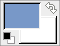Filter Border Average applied

This plug-in calculates the most often used color in a specified border of the active layer or selection. It can gather similar colors together so that they become predominant. The calculated color becomes the foreground color in the Toolbox. This filter is interesting when you have to find a Web page color background that differs as little as possible from your image border. The action of this filter is not registered in Undo History and can't be deleted with Ctrl+Z : it doesn't modify the image.

#### 8.29.2. Activating the filter

This filter is found in the image window menu under Colors+InfoBorder Average.

#### 8.29.3. Options

Figure 16.181. Options of the Border Average Filter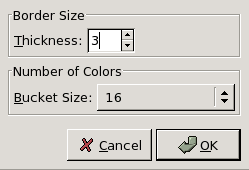Border Size

You can set there the border Thickness in pixels.

Number of Colors

The Bucket Size lets you control the number of colors considered as similar and counted with the same bucket. A low bucket size value (i.e. a high bucket number) gives you better precision in the calculation of the average color. Note that better precision does not necessarily mean better results (see example below).

#### 8.29.4.  Examples illustrating the “Border Average” filter

Figure 16.182. Original image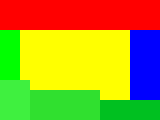Original image: colors are pure Red (255;0;0), pure Blue (0;0;255), and different but similar kinds of Green ( 0;255;0 , 63;240;63 , 48;224;47 , 0;192;38 ).

Figure 16.183. Number of Colors is set to 8: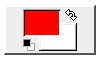The resulting color is a Red (254,2,2).

The bucket size is low. So the bucket number is high. All color shades can be stored in different buckets. Here, the bucket containing red is the most filled. The resulting color is a nearly pure Red (254,2,2) and becomes the foreground color of the Toolbox.

Figure 16.184. Number of Colors is set to 64: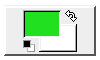The resulting color is Green (32,224,32).

Here the bucket size is high, the number of buckets low. Similar colors (here green) are stored in a same bucket. This green bucket is now the most filled. All colors in this bucket have the same values for the two most significant bits: (00******;11******;00******). The remaining 6 bits may have any values from 0 to 63 for the respective channel. So in this bucket, color red channels range from 0 to 63, green channels from 192 to 255, blue channels from 0 to 63. The resulting color is Green (32,224,32), which, for every channel, is the average between the limits of the channel range (63 + 0)/2, (255+192)/2 , (63+0)/2.